# How to add a lot of Goal Seeking to a model

Huis , Posts by Hui , simulation - 10 comments

Recently in the Chandoo.org Forums, MR06 asked the question, “I am trying to create a sensitivity table that tells me what amount of equity I need to include in a deal in order to get a fixed IRR as the year 3 sales price changes.

MR06 was using Goal Seek to change the equity input so that the IRR achieved 25% value. But he wanted to do that for a number of Year 3 price changes in the model.

He could obviously change the Year 3 value manually, then run a Goal Seek and write down the Solution and repeat for the range of Year 3 prices changes.

Or he could have setup a macro to do the same and tabulate the results.

Eventually I provided a solution which uses a Data Table and some formulas to determine these values

This post will examine the technique used to solve the problem for MR06

## The Problem

This is MR06’s model. It is a simple financial model of an investment in some units over a 6 1/2 year period.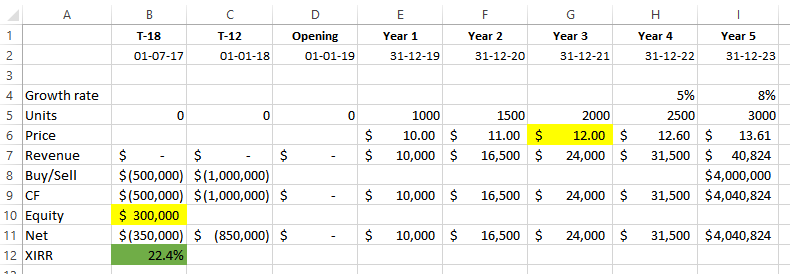The problem is that MR06 wants to know what Equity value B10 is required to achieve an Internal Rate of Return (IRR) of 25% subject to varying the Year 3 Price, cell G6.

## Goal Seek

This problem can be solved using Goal Seek
Goto the Data, What-If-Analysis, Goal Seek TabSet the dialogs in the Goal Seek to the values shown above and press Ok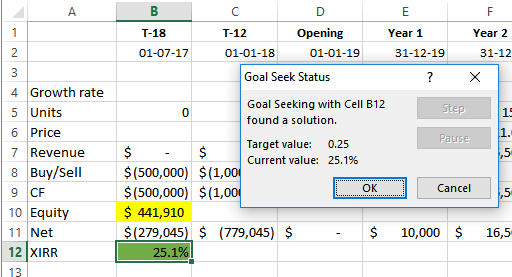Excel sets the value in cell B12 to the value of \$441,910 and shows an IRR of 25.1%. This small discrepancy will be discussed later.

MR06 can now manually repeat that changing the values of G6 between each iteration. Except that MR06 has many many models and wants to do that for variations in the Year 3 Price from \$8 to \$15.

## Data Tables

An alternative method to solve this is to use a Data Table to feed in the starting values for both the Year 3 Price G6, as well as a list of Values for the Equity Contribution B12.

The Data Table will then retrieve a list of the IRR’s for the various combinations.

Then we can then setup a formula to calculate the Equity contribution required to achieve the target IRR value of 25%

### Firstly setup a Data Table

Setup a list of required Prices for Year 3 in a Row D15:K15 and a list of possible Equities in a Column C16:C25I have colored the cells to simplify which cells are linked to which parts of the Data Table.

In the Top Left cell C15 link that to the Target IRR cell using =B12Now select the whole area C15:K25 and goto the Data, What-If-Analysis, Data Table Tab

Complete the Data Table dialog
Row Input cell:  \$G\$6
Row Input cell:  \$B\$10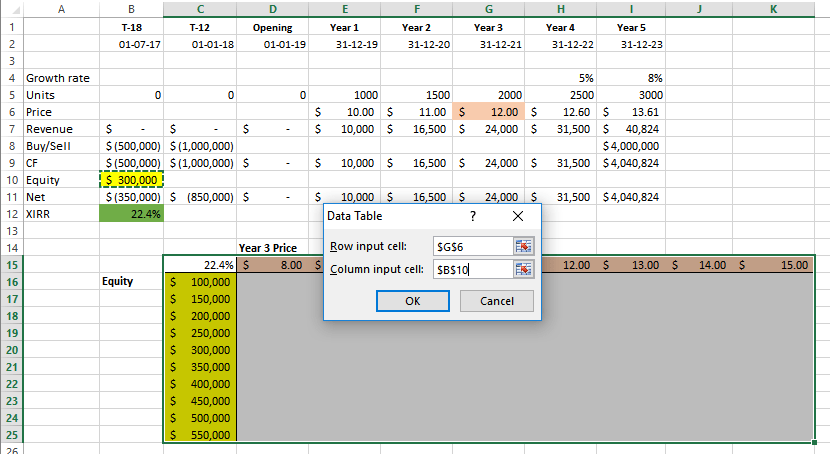Press Ok

Excel will now fill in the Data Table area with the IRR’s from the combination of Each Row (Equity) and Column (Year 3 Price)At this point we now have a table of IRR’s from the combination of Each Row (Equity) and Column (Year 3 Price), but we can see that we don’t have an actual solution, only approximate solutions for each Year 3 Price.

Our goal was to find the Equity value which returned a 25% IRR

We can use the Excel Forecast() function to interpolate the exact answers based on the data in the Data Table

Forecast allows us to forecast a Y value based on an X value from a Table of Known X and Y valuesWe can see from the help that Forecast uses the syntax:
=FORECAST(x, known_y’s, known_x’s)
In our example we are seeking a known value of the IRR of 25%
We have a List of Known X’s which is the IRR’s in the Data Table and the list of known Y’s is the corresponding list of Equities in Column C

To test this in cell D27 type the following: =FORECAST(25%,\$C16:\$C25,D16:D25) and press Enter
Excel will return \$444,481.25This is the equity required to return 25% IRR based on a Year 3 Price of \$8.00

You can copy Cell D27 across and you will now have a Table of all the equities required for all Year 3 Prices.A neater solution is to also allow the IRR to be set as a variable. This way it can be changed and the new equities automatically shown for each Year 3 Price change
In Cell D29 type 25%
Then setup a Row of Year 3 Prices in cell D31 type: =D15
Copy D31 across to K1
Then in D32 type: =FORECAST(\$D\$29,\$C16:\$C25,D16:D25)
Copy it across to K32

You now have a table of Year 3 Prices and the required Equities to achieve a 25% IRR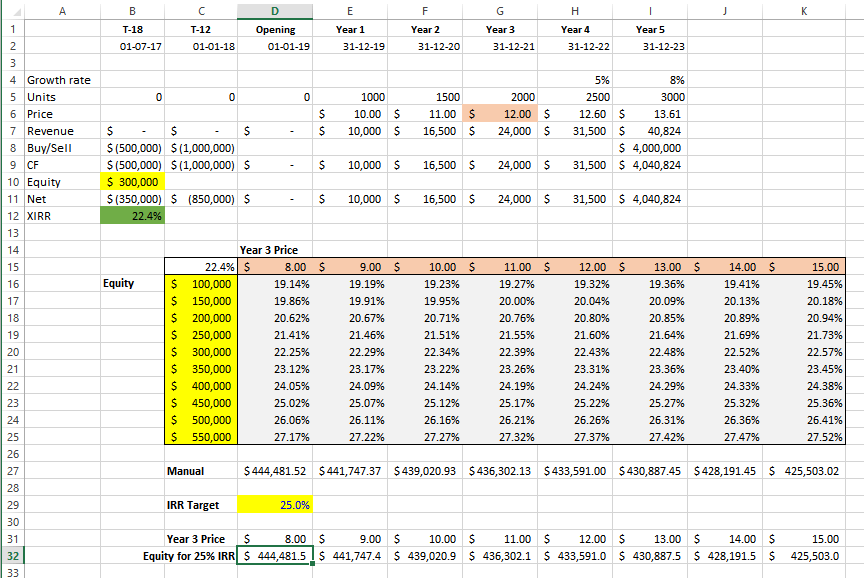You can simply change the Required IRR in Cell D29 eg 20%
And you will have a Table of the required Equities to achieve a 20% IRR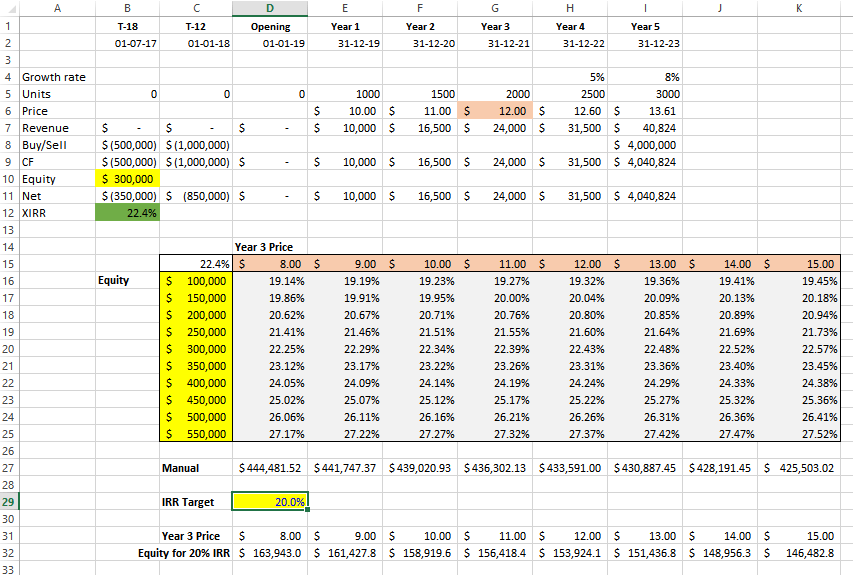The good thing about having this as a separate table is that it can easily be graphed
Select the Table and got the Insert, Chart Tab
Select an appropriate chart type### One warning

In this example the model appears to be very linear, this means there is a direct or straight line relationship between the Equity and the IRR for a given Year 3 Price

If we choose a value of equity of say 30% we can see that we need an equity of \$725,020 for a Year 3 Price of 8.00It is important to note that the value of \$725,020 is outside the range of the Equity values we set in Column C

In this case we should check that this is still a valid Forecast

To do this reset the Equity values in Column C to that shown below, ensuring that the equity values extend both below and above the expected equity value we saw of \$725,020We can now see that the Equity value required is \$649,577.

### Warning:

Those who are observant will notice that the Equity value of 649,577 should have an IRR of slightly less than 29.61%, not 30%

This is because although the model appears linear, it is in fact not exactly linear.

The model’s non-linearity occurs for a number of reasons but primarily is that XIRR is not an exact function. It is an Iterative Function and to quote from Microsoft Help “Excel uses an iterative technique for calculating XIRR. Using a changing rate (starting with guess), XIRR cycles through the calculation until the result is accurate within 0.000001 percent.

For all intention purposes it is close enough to linear that we don’t notice the differences.

If it was perfectly linear the extrapolation to 30% which was outside the original Equity range would have given us the same result of \$649,577 not the \$725,020 that it returned. Non-linearity is magnified once the extrapolation used in the Forecast Function is outside the range of the known Y values and we need to always check for this.

This non-linearity causes small discrepancies in the Excel functions like IRR and Forecast, of which we are using both.

Forecast uses a least squares approximation to best estimate a line of best fit, but it is just that, an approximation.

If you are wondering how close the model is to being linear, it is 99.77% fit to being linear

This is calculated using the r value or Pearson() function
D50: =PEARSON(C16:C25,D16:D25)
=0.997747518
That is the closer the value is to 1 the more linear it is.

### Excel 2016+

In Excel versions 2016+ Microsoft has added a new set of functions including: FORECAST.LINEAR()

Forecast.Linear and the other new Statistical Functions “use advanced machine learning algorithms, such as Exponential Triple Smoothing (ETS)

It is recommended that they are used in model from using Excel 2016 onwards.

## Closing

I hope you have enjoyed this discussion on the use of Data Tables and the Forecast() Function to replicate multiple Goal Seek commands.

For more information on Data Tables I refer you to my post: Data Tables & Monte Carlo Simulations in Excel – A Comprehensive Guide

Many thanx to MR02 for permission to use his model for this post.Hello Awesome...

My name is Chandoo. Thanks for dropping by. My mission is to make you awesome in Excel & your work. I live in Wellington, New Zealand. When I am not F9ing my formulas, I cycle, cook or play lego with my kids. Know more about me.

Thank you and see you around.

### Related articles:

 Written by Hui... Tags: Forecast, Pearson(), xirr() Home: Chandoo.org Main Page ? Doubt: Ask an Excel Question

### 10 Responses to “How to add a lot of Goal Seeking to a model”

1.Rocky_Rocks says:

Thanks for this, though the data table with goal seeked values could be achieved easily through a simple macro using loops without having the problem of linearity.

2.prashant says:

3.Chrojas says:

This was such a good setup of a problem with a terrible solution.

Several key points:
1. The math is such that an IRR calculation is done iteratively. That's why you're allowed to submit a guess. The format of the IRR function is: IRR(values, [guess]) . I'm not aware of any other functions which take a guess, except...
2. XIRR - should always and everywhere be used. Never, ever, ever use the IRR function. It's a receipe for disaster. Your spreadsheet shows this but the point should be explicit.
3. If you're calculating an IRR or an XIRR, it's because you're making an investment decision. And more likely than not, if you're a spreadsheeter and you're calculating an IRR or XIRR, you're calculating a return on somebody else's money. So do yourself a favor and take the time to do it properly. Actually calculate it. Don't take shortcurts.

What this means is USE THE XIRR FUNCTION.

4. Don't assume that things are linear. They might not be. Don't calculate a few points and then use FORECAST to fill in the rest. That's a sure fire way to get yourself fired or lose money. If an analyst handed me a spreadsheet that used FORECAST in it for an IRR I would tell them to take the time to redo the analysis properly, or find a new job.

5. The right way to calculate a bunch of IRRs is to automate things with a macro. A simple goal seek has three variables. You can simplify it down to two by always backsolving to zero with one line of VBA and a couple of range names.

Range("target").GoalSeek Goal:=0, ChangingCell:=Range("change")

Wrapping this into a loop to fill out a sensitivity table is not too hard, and it would be worthy of a followup post.

•Hui... says:

@Chrojas

The post was written as an example of using Data Tables and Forecast to perform multiple Goal Seek functions within a model.

Nowhere in the post do I say use either IRR() or XIRR().
Each can be used to give an Internal Rate of Return within the limitations of the function and the suiability & limitations of your model.

The model specifically identifies that it uses XIRR and uses XIRR in the calculations.

The errors are not introduced by the Forecast function but come from the errors in the XIRR function.

Using XIRR with a poor guess can give widely differing and yet all correct answers for nonlinear problems.
Refer how XIRR can return 3 results: https://freefincal.com/irr-xirr-excel-limitations-calculate-returns/

The use of Goal-Seek is also an approximation tool and does return the correct answer, as highlighted in the post it returns 25.1%IRR, Third image above, so using that in a loop in a macro won't fix that issue. In fact there is a better case for writing a follow up warning post on the use of Goal Seek with XIRR as the two iterative functions together probably shouldn't be practised.

Using approximation tools such as Forecast() is an acceptable method of finding IRR's: http://www.corality.com/tutorials/calculate-irr-excel

•MR06 says:

Hi Chrojas,

With regards to my specific issue, which requires 2 steps (1: set the year 3 price, 2: goal seek the equity to achieve 25% IRR), how would you set up that macro? The end goal is to create rows 31 and 32 in Hui's example, but in a quick, repeatable way such that I can copy/paste with minimal adjustments to solve this question for hundreds of different investments.

The real models used are significantly more complex than this, although they do have simple inputs for price and equity.

Thanks!

MR06

•Stan Bown says:

Have you got a macro solution you can share?

4.John MacDougall says:

Just a note about why the IRR calculation involves an iteration rather than an exact solution. IRR essentially is finding the roots of a polynomial equation. There are closed form algebraic solutions when the highest power in the polynomial is 4 or less but no closed form solutions exist when the highest power is 5 or more.

You probably remember the closed form solution for a polynomial of power 2:

(-b+-root(b^2-4ac))/2a

The solution for 3 and 4 get pretty ugly and there are no general solutions for 5 and over (although there are solutions for special cases).

5.Bob says:

Many thanks Hui!

Despite Chrojas' moaning I found this very useful

6.Hassan says:

7.Stan Bown says:

Very useful, all round. Thanks to question, answer and commentators.

 « Rounding time to nearest minute or quarter hour etc. [formulas] Joyplot in Excel »

### Get FREE Excel & Power-BI Newsletter

One email per week with Excel and Power BI goodness. Join 100,000+ others and get it free.